# Binary Codes

In the coding, when numbers, letters or words are represented by a specific group of symbols, it is said that the number, letter or word is being encoded. The group of symbols is called as a code. The digital data is represented, stored and transmitted as group of binary bits. This group is also called as binary code. The binary code is represented by the number as well as alphanumeric letter.

Following is the list of advantages that binary code offers.

• Binary codes are suitable for the computer applications.

• Binary codes are suitable for the digital communications.

• Binary codes make the analysis and designing of digital circuits if we use the binary codes.

• Since only 0 & 1 are being used, implementation becomes easy.

## Classification of binary codes

The codes are broadly categorized into following four categories.

• Weighted Codes
• Non-Weighted Codes
• Binary Coded Decimal Code
• Alphanumeric Codes
• Error Detecting Codes
• Error Correcting Codes

## Weighted Codes

Weighted binary codes are those binary codes which obey the positional weight principle. Each position of the number represents a specific weight. Several systems of the codes are used to express the decimal digits 0 through 9. In these codes each decimal digit is represented by a group of four bits.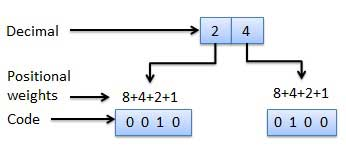## Non-Weighted Codes

In this type of binary codes, the positional weights are not assigned. The examples of non-weighted codes are Excess-3 code and Gray code.

### Excess-3 code

The Excess-3 code is also called as XS-3 code. It is non-weighted code used to express decimal numbers. The Excess-3 code words are derived from the 8421 BCD code words adding (0011)2 or (3)10 to each code word in 8421. The excess-3 codes are obtained as follows −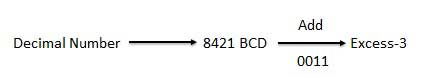### Example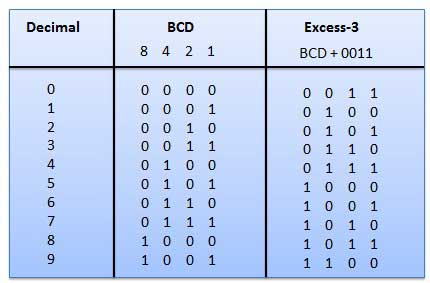### Gray Code

It is the non-weighted code and it is not arithmetic codes. That means there are no specific weights assigned to the bit position. It has a very special feature that, only one bit will change each time the decimal number is incremented as shown in fig. As only one bit changes at a time, the gray code is called as a unit distance code. The gray code is a cyclic code. Gray code cannot be used for arithmetic operation.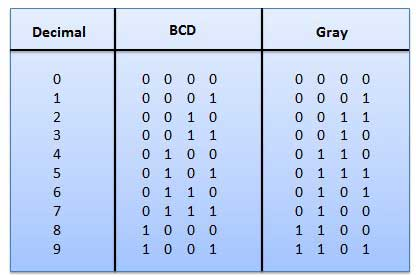### Application of Gray code

• Gray code is popularly used in the shaft position encoders.

• A shaft position encoder produces a code word which represents the angular position of the shaft.

## Binary Coded Decimal (BCD) code

In this code each decimal digit is represented by a 4-bit binary number. BCD is a way to express each of the decimal digits with a binary code. In the BCD, with four bits we can represent sixteen numbers (0000 to 1111). But in BCD code only first ten of these are used (0000 to 1001). The remaining six code combinations i.e. 1010 to 1111 are invalid in BCD.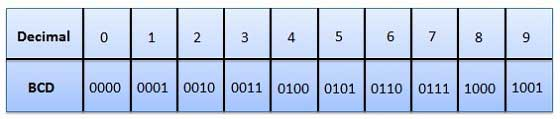• It is very similar to decimal system.
• We need to remember binary equivalent of decimal numbers 0 to 9 only.

• The addition and subtraction of BCD have different rules.

• The BCD arithmetic is little more complicated.

• BCD needs more number of bits than binary to represent the decimal number. So BCD is less efficient than binary.

## Alphanumeric codes

A binary digit or bit can represent only two symbols as it has only two states '0' or '1'. But this is not enough for communication between two computers because there we need many more symbols for communication. These symbols are required to represent 26 alphabets with capital and small letters, numbers from 0 to 9, punctuation marks and other symbols.

The alphanumeric codes are the codes that represent numbers and alphabetic characters. Mostly such codes also represent other characters such as symbol and various instructions necessary for conveying information. An alphanumeric code should at least represent 10 digits and 26 letters of alphabet i.e. total 36 items. The following three alphanumeric codes are very commonly used for the data representation.

• American Standard Code for Information Interchange (ASCII).
• Extended Binary Coded Decimal Interchange Code (EBCDIC).
• Five bit Baudot Code.

ASCII code is a 7-bit code whereas EBCDIC is an 8-bit code. ASCII code is more commonly used worldwide while EBCDIC is used primarily in large IBM computers.

## Error Codes

There are binary code techniques available to detect and correct data during data transmission.

Error Code Description

Error Detection and Correction

Error detection and correction code techniques